# Seven Steps to Successful Analog-to-Digital Signal Conversion (Noise Calculation for Proper Signal Conditioning)

High precision applications require a well-designed low noise analog front end to get the best SNR, which requires an informed approach to choosing an ADC to fully and accurately capture sensor signals. Support components such as driver op amps and references are selected to optimize overall circuit performance.

Real-world signals, such as vibration, temperature, pressure, and light, require accurate signal conditioning and signal conversion before further data processing in the digital domain. In order to overcome many challenges in today’s high precision applications, a well-designed low noise analog front end is needed to get the best SNR. Many systems cannot afford the most expensive parts, nor can they afford the higher power consumption of low noise parts. This article addresses questions about designing a total solution using a noise-optimized approach. This article presents a methodical approach to the design of a gain block and ADC combination, including an example that supports this approach. Noise calculation and analysis is performed on this circuit when conditioning low frequency (near dc) signals.

Follow these seven steps when designing an analog front end:

1. Describe the electrical output of the sensor or section preceding the gain block.
3. Find the optimal ADC + voltage reference for the signal conversion.
4. Find the maximum gain and define search criteria for the op amp.
5. Find the optimal amplifier and design the gain block.
6. Check the total solution noise against the design target.
7. Run simulation and validate.

### Step 1: Describe the electrical output of the sensor or section preceding the gain block

Signals can come directly from the sensor or may have gone through EMI and RFI filters prior to the gain block. In order to design the gain block, one needs to know the ac and dc characteristics of the signal and the available power supplies. Knowing the signal’s characteristic and noise level provides clues as to what input voltage range and noise levels we might need when selecting an ADC. Let’s assume that we have a sensor that outputs a 10 kHz signal with full-scale amplitude of 250 mV p-p (88.2 mV rms) and 25 μV p-p noise. Let’s additionally assume that we have a 5 V supply available in our system. With this information we should be able to calculate the signal-to-noise ratio at the ADC’s input in step 2. To simplify data crunching and confusion, assume that we design this solution for room temperature operation.

### Step 2: Calculate the ADC’s requirement

What type of ADC, what sample rate, how many bits, and what noise specification do we need? By knowing the input signal amplitude and noise information from step 1, we can calculate the signal-to-noise ratio (SNR) at the gain block’s input. We need to pick an ADC that has better signal-to-noise ratio. Knowing the SNR will help us to calculate the effective number of bits (ENOB) when choosing the ADC. This relationship is shown in the following equations. Both SNR and ENOB are always specified in any good ADC data sheet. In this example, the required 86.8 dB SNR and 14.2-bit ENOB force us to choose a 16-bit analog-to-digital converter. Additionally the Nyquist criterion states that the sampling rate, fs, should be at least twice the maximum incoming frequency, fin, so a 20 kSPS ADC would suffice.

Next, we need to design an overall solution with a noise density that does not exceed 416 nV/√Hz. This places the noise of the signal conditioning circuit at 1/10 of the input noise.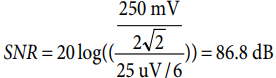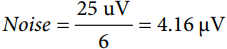### Step 3: Find the optimal ADC + voltage reference to do the signal conversion

Having a set of search criteria on hand, there are many ways to find the ADC that can fit the requirements. One of the easiest ways to find a 16-bit ADC is to use the search tool on the manufacturer’s site. By entering resolution and sample rate, a number of choices are suggested.

Many 16-bit ADCs specify 14.5 bits of ENOB. If you would like to have better noise performance, use oversampling to push the ENOB up to 16 bits (n-bit improvement is obtained from 4n oversampling). With oversampling, one could use a lower resolution ADC: a 12-bit ADC oversampled by 256 (44 oversampling) will yield 16-bit noise performance. In our example, this means a 12-bit ADC with 5.126 MHz sample rate (20 kSPS × 256). Or, a 14-bit ADC oversampled by 42; or 1.28 MSPS might be better. These cost as much, however, as the AD7685 16-bit, 250 kSPS ADC.

The AD7685 16-bit PulSAR® ADC is selected from the list. This converter has 90 dB SNR and 250 kSPS sample rate to suit our requirements. The ADR421/ADR431 precision XFET® voltage references are recommended for use with this ADC. The 2.5 V input range exceeds our 250-mV p-p input specification.The AD7685’s reference input has dynamic input impedance, so it should be decoupled with minimal parasitic inductances by placing a ceramic decoupling capacitor close to the pins and connecting it with wide, low impedance traces. A 22 μF ceramic chip capacitor will provide optimum performance.

### Step 4: Find the maximum gain and define search criteria for the op amp

Knowing the input voltage range of the ADC will help us in designing the gain block. To maximize our dynamic range, we need to take the highest gain possible with the given input signal and ADC’s input range. This means that we can design our gain blocks to have a gain of 10 for the example on hand.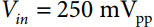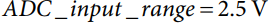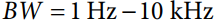Although the AD7685 is easy to drive, the driver amplifier needs to meet certain requirements. For example, the noise generated by the driver amplifier needs to be kept as low as possible to preserve the SNR and transition noise performance of the AD7685, but remember that the gain block amplifies both signal and noise together. To keep the noise at the same level before and after the gain block, we need to select an amplifier and components that have much lower noise. The driver should also have THD performance commensurate with the AD7685 and must settle a full-scale step onto the ADC’s capacitor array at a 16-bit level (0.0015%). The noise coming from the amplifier can be further filtered by an external filter.

How much noise is allowed at the input of the opamp? Remember that we need to design an overall solution whose noise density does not exceed 416 nV/rt-Hz. We should design a gain block that has much lower noise floor, say by a factor of 10 since we gain up by 10. This will ensure that noise from amplifier is much less than the noise floor of the sensor. To calculate the noise margin, we can roughly assume that the noise at the input of the op amp is the total noise of the op amp plus the noise of the ADC.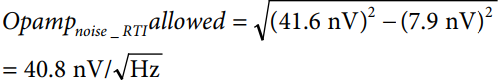### Step 5: Find the best amplifier and design the gain block

The first order of op amp selection after knowing the input signal bandwidth is to pick an op amp that has an acceptable gain-bandwidth product (GBWP) and that can process this signal with minimum amount of dc and ac errors. To get the best gain bandwidth product, the signal bandwidth, noise gain, and gain error are required. These terms are all defined below. As a guide, pick an amplifier that has gain bandwidth greater than 100 times the input signal BW if you want to keep the gain error below 0.1%. Additionally, we need an amplifier that settles quickly and has good drive capability. Remember that our noise budget requires the overall noise at the input of the op amp to be less than 40.8 nV/√Hz, while the ADC specifies 7.9 nV/√Hz. To summarize the search criteria for the op amp: UGBW > 1 MHz, single 5 V supply, good voltage noise, current noise, and THD specs, low dc errors not to degrade ADC’s specs.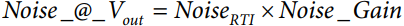Using a similar approach to the ADC search, the AD8641 is picked for our example. The AD8641 low power, precision JFET input amplifiers feature extremely low input bias current and rail-to-rail output that can operate with supplies of 5 V to 26 V. Its relevant specs are stated in the table below. We can configure the op amp in a noninverting configuration with the component values shown in the table.

 Component Value R1 1.47 kΩ R2 13.3 kΩ R3 1.47 kΩ En 28.5 nV/√Hz In 50 fA/√Hz Cf 0.47 nF

All active and passive components generate noise of their own, so it is important to choose components that do not degrade performance. As an example, it is wasteful to buy a low noise op amp and surround it with large resistors. Remember that a 1-kΩ resistor has 4 nV of noise.

As mentioned earlier, an optional RC filter can be used between the ADC and this gain block, which should help in narrowing BW and improving SNR.

### Step 6: Check total solution noise against your design targets

It is extremely important to have a good understanding of all the error sources in the designed circuit. In order to achieve the best SNR, we need to write out the overall noise equation for the above solution. This is shown in the equation below.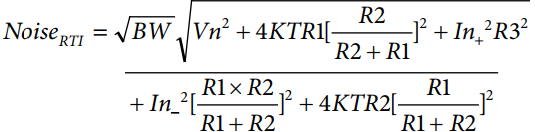We can calculate the total noise at the input of the op amp and make sure that it is less than the 41.6 nV/√Hz as we had planned.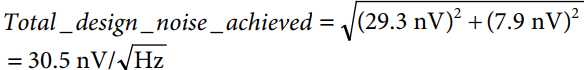To integrate the total noise over the entire bandwidth, we can see that the total noise at the input of the ADC over the filter’s bandwidth is 3.05 μV, which is less than the 4.16 μV requirement of our design. The low frequency noise (1/f) is ignored in this case since the corner frequency of the AD8641 is below 100 Hz.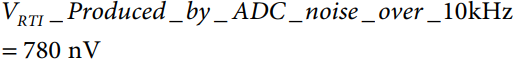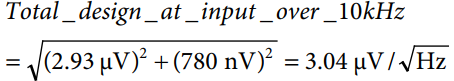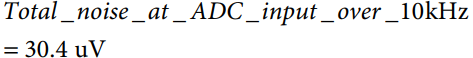Maintaining a good signal-to-noise ratio requires paying attention to the noise of every element in the signal path and good PCB layout. Avoid running digital lines under any ADC because these couple noise onto the die, unless a ground plane under the ADC is used as a shield. Fast switching signals, such as CNV or clocks, should never run near analog signal paths. Crossover of digital and analog signals should be avoided.

### Step 7: Run simulation and validate

Using PSpice Macro-models, downloadable from the ADI site, can be a good starting point for validation of any circuit design. A quick simulation shows the signal bandwidth for which we designed our solution. Figure 4 shows the response before and after the optional RC filter at the input of the AD7685.

As shown in Figure 5, the total output noise over the 10 kHz bandwidth is close to 31 μV rms. This is less than the design target of 41 μV rms. Bench prototypes needs to be built, and the whole solution has to get validated before full production.Figure 5. Simulation for noise response of circuit in Figure 3.

### Summary

With today's low power, cost conscious designs, many systems cannot afford the most expensive parts, nor can they afford the higher power consumption of low noise parts. To attain the lowest noise floor and best performance from signal conditioning circuitry, designers must understand component level noise sources. Maintaining a good signal-to-noise ratio requires attention to the noise of every element in the signal path. By following the above steps, one can successfully condition a small analog signal and convert it using a very high resolution ADC.

### Ссылки

4. Seminar: Noise Optimization in Sensor Signal Conditioning Circuits, Part 1.

5. Seminar: Noise Optimization in Sensor Signal Conditioning Circuits, Part 2.